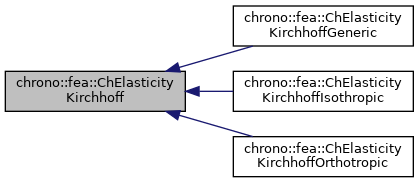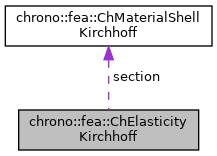chrono::fea::ChElasticityKirchhoff Class Referenceabstract

## Description

Base interface for elasticity of thin shells (Kirchoff-Love shell theory, without shear effects) to be used in a ChMaterialShellKirchhoff.

Children classes must implement the ComputeStress function to get {n,m}=f({e,k}) that is per-unit-length forces/torques n_11,n22,n12,m_11,m_22,m_12 given the strains/curvatures e_11 e_22 e_12 k_11 k_22 k_12. Inherited materials do not define any thickness, which should be a property of the element or its layer(s) using this material.

#include <ChMaterialShellKirchhoff.h>

Inheritance diagram for chrono::fea::ChElasticityKirchhoff:[legend]
Collaboration diagram for chrono::fea::ChElasticityKirchhoff:[legend]

## Public Member Functions

virtual void ComputeStress (ChVector<> &n, ChVector<> &m, const ChVector<> &eps, const ChVector<> &kur, const double z_inf, const double z_sup, const double angle)=0
Compute the generalized force and torque, given actual deformation and curvature. More...

virtual void ComputeStiffnessMatrix (ChMatrixRef mC, const ChVector<> &eps, const ChVector<> &kur, const double z_inf, const double z_sup, const double angle)
Compute the 6x6 stiffness matrix [Km] , that is [ds/de], the tangent of the constitutive relation stresses/strains. More...

## Public Attributes

ChMaterialShellKirchhoffsection

## ◆ ComputeStiffnessMatrix()

 void chrono::fea::ChElasticityKirchhoff::ComputeStiffnessMatrix ( ChMatrixRef mC, const ChVector<> & eps, const ChVector<> & kur, const double z_inf, const double z_sup, const double angle )
virtual

Compute the 6x6 stiffness matrix [Km] , that is [ds/de], the tangent of the constitutive relation stresses/strains.

By default, it is computed by backward differentiation from the ComputeStress() function, but inherited classes should better provide an analytical form, if possible.

Parameters
 mC tangent matrix eps strains e_11, e_22, e_12 kur curvature k_11, k_22, k_12 z_inf layer lower z value (along thickness coord) z_sup layer upper z value (along thickness coord) angle layer angle respect to x (if needed)

## ◆ ComputeStress()

 virtual void chrono::fea::ChElasticityKirchhoff::ComputeStress ( ChVector<> & n, ChVector<> & m, const ChVector<> & eps, const ChVector<> & kur, const double z_inf, const double z_sup, const double angle )
pure virtual

Compute the generalized force and torque, given actual deformation and curvature.

This MUST be implemented by subclasses.

Parameters
 n forces n_11, n_22, n_12 (per unit length) m torques m_11, m_22, m_12 (per unit length) eps strains e_11, e_22, e_12 kur curvature k_11, k_22, k_12 z_inf layer lower z value (along thickness coord) z_sup layer upper z value (along thickness coord) angle layer angle respect to x (if needed)

The documentation for this class was generated from the following files:
• /builds/uwsbel/chrono/src/chrono/fea/ChMaterialShellKirchhoff.h
• /builds/uwsbel/chrono/src/chrono/fea/ChMaterialShellKirchhoff.cpp